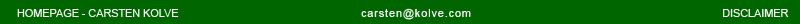MAYA, TUTORIALS, ROLLING ELLIPSE PT.2 - WACKY VEHICLE

ABOUT
While you have learned in the rolling ellipse tutorial how to roll an ellipse on the floor, we will now use this expression to build a funny moving vehicle. As I am no artist I won't focus on modelling issues, but on the math to make this little thingy move.

MODELING
Let's build a simple proxy model of a vehicle. We'll start with the wheels: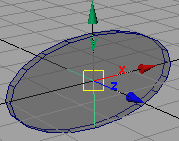Create a polygon cylinder (radius = 1.0; height = 0.3; subDivs around axis = 20; subdivs on caps = 0; axis = z;), rename it to "wheelFL" - this will be our front-left wheel. Delete its history. Scale the wheel by 2.0 in x-direction. Create a locator "locFL", make it a child of the wheel. This will be the contact point with the main body later on. Note: Of course you are free to use a nurbsCylinder, too.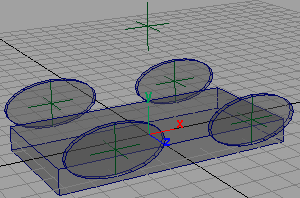Dublicate the wheel 3 times. Rename the new wheels to "wheelFR", "wheelBL", "wheelBR". Update the child locators accordingly, too. Move "wheelFL" to [3,1,-3], "wheelFR" to [3,1,3], "wheelBL" to [-3,1,-3], "wheelBR" to [-3,1,3]. Create a polyCube "body" (w=10; h=1;d=5). Create a locator "vehicle", move it above the vehicle parts and freeze its transforms.

RIGGING
Now that we have build ourselfs a nice little vehicle, let's add some funcionality.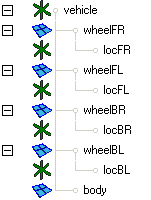First, parent all vehicle parts to the "vehicle" locator. We will use this locator to move the vehicle later on. Your outliner should look like this: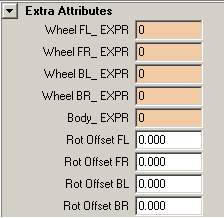We will now turn the "vehicle" locator into the interface to the expressions, driving the animation of our vehicle. Add custom attributes as shown on the left to the "vehicle" node. The ones ending with "_EXPR" are of type "integer" and their purpose is to give us easy access to the respective expression. The other ones will serve us as a means to enter a rotational offset for each wheel. Ther are of type "float".

Now we'lll add slightly modified version of the roll ellipse script to each of the wheels.
Add this expression to each of the respective wheel expression interface attributes, don't forget to change the name of the wheel accordingly (you can quickly do this by using a "search and replace" on "FL" in your favourite text editor). I won't go much into details here, take a look at the first tutorial for more information.

 // helper connection to the interface attribute vehicle.wheelFL_EXPR =0; // constant values \$pi = 3.141592; // radii of the elliptical wheel \$rA = 2.0; \$rB = 1.0; // get translation of the vehicle and offset of the wheel \$x = vehicle.translateX; \$rotZOffset = vehicle.rotOffsetFL; // as we are working in radians, convert the rot offset \$rotZOffset = deg_to_rad(\$rotZOffset); // compute perimeter of elliptical wheel \$per = \$pi*(\$rA+\$rB); // compute the rotation of the wheel \$rot = (-2*\$pi*\$x/\$per)+\$rotZOffset; // adjust the y-position of the wheels center \$y = abs((-sin(\$rot)*tan(\$rot)*\$rA*\$rA)-(cos(\$rot)*\$rB*\$rB))/      sqrt(\$rA*\$rA*pow(tan(\$rot),2)+\$rB*\$rB); // apply the results to our wheel wheelFL.translateY = \$y; wheelFL.rotateZ = rad_to_deg(\$rot);

Test if all the wheels are rolling correctly by moving the vehicle locator in x-direction. Change the rotational offset values to test if they work correctly, too. Make sure everything runs fine.
OK? Good, on to the movement of the body. The pupose of our expression is to move the main body so it tries to stay in contact with the contact locators on the wheels. Our first concern is to adjust the position of the main body, we will move this to the mean position of all contact locators. Of course, we've got to use their world position for this as they are not on the same hirachy level as our "body"!

 // helper connection to the interface attribute vehicle.body_EXPR =0.0; // get positions of contact points of every wheel float \$pos[]; \$pos = `xform -q -ws -t locFL`; vector \$posFL = <<\$pos,\$pos,\$pos>>; \$pos = `xform -q -ws -t locFR`; vector \$posFR = <<\$pos,\$pos,\$pos>>; \$pos = `xform -q -ws -t locBL`; vector \$posBL = <<\$pos,\$pos,\$pos>>; \$pos = `xform -q -ws -t locBR`; vector \$posBR = <<\$pos,\$pos,\$pos>>; // compute mean position vector \$meanPos = (\$posFL + \$posFR + \$posBL + \$posBR) / 4.0; // [..]

Our next step is to get the rotation in x- and z-direction for our body. We'll measure the angles between the relative axis and the vector between the respective wheels, to obtain the angle by which we have got to rotate the vehicle's body.

 // [..] // get x-angle between the left and right contact position and // the z-axis, do this for front and back, our rotation angle // will be the mean angle float \$angleXF = `angle <<0.0,0.0,1.0>> ( \$posFL - \$posFR )`; if (\$posFL.y > \$posFR.y) \$angleXF = -\$angleXF; float \$angleXB = `angle <<0.0,0.0,1.0>> ( \$posBL - \$posBR )`; if (\$posBL.y > \$posBR.y) \$angleXB = -\$angleXB; float \$angleX = (\$angleXB+\$angleXF)/2.0; // get z-angle between the front and back contact position and // the x-axis, do this for left and right, our rotation angle // will be the mean angle float \$angleZL = `angle <<1.0,0.0,0.0>> ( \$posFL - \$posBL )`; if (\$posFL.y < \$posBL.y) \$angleZL = -\$angleZL; float \$angleZR = `angle <<1.0,0.0,0.0>> ( \$posFR - \$posBR )`; if (\$posFR.y < \$posBR.y) \$angleZR = -\$angleZR; float \$angleZ = (\$angleZL+\$angleZR)/2.0; // [..]

Finally, we have got to apply the results to the "body":

 // [..] // apply results to body, take into account that "body" is // parented to "vehicle" body.translateX = \$meanPos.x - vehicle.translateX; body.translateY = \$meanPos.y - vehicle.translateY; body.translateZ = \$meanPos.z - vehicle.translateZ; body.rotateX = rad_to_deg(\$angleX); body.rotateZ = rad_to_deg(\$angleZ);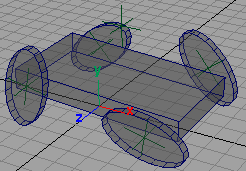Here you are! Some suggestions: - try different rotational offset settings - change the size of the wheels (don't forget to adjust the radii in the expressions!) - move the position of the contact locators on the wheels

EXAMPLE MOVIE
Watch an example movie with the vehicle in action > wackyVehicle.avi (xxx mb) (todo)

DOWNLOAD
example scene and expressions > rollEllipse1_1.zip
(located at highend3d.com)